# Mathematical Induction

The journey of a thousand miles starts with a single step.

Lao Tzu

Many statements in mathematics are true {\em for any natural number}. For example.

Fundamental Theorem of Arithmetic. Every natural number has a unique prime decomposition.

Theorem. Every natural number is either even or odd.

Power Rule for Differentiation. For any natural number,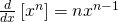.

Our first use of the definition of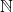(other than to prove facts about arithmetic) will be to establish a way to prove statements like this. But to do that, we need to make sure our definition ofis formal enough to serve as the backbone of a proof. To do this, we'll focus on the structure of the open sentences involved. Since we are interested only in statements that involve the natural numbers somehow, we're going to assume that the variables we're dealing with represent natural numbers unless otherwise specified. That is, we're taking the universe for everything to beuntil further notice.

Definition. We call an open sentence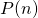inductive if it has the property: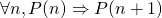.

We can think of inductive sentences as representing hereditary properties -- that is, they pass down to successors. Being royal, for example, is an inductive property.

Equipped with this definition, we can state axiom 5 of the natural numbers as a formal symbolic sentence. (If you recall, we stated the first four axioms as formal symbolic sentences, but left the fifth axiom just in terms of words.) Here goes:

The Inductive Axiom. Letbe an open sentence whose variablerepresents a natural number. Suppose that:

•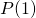is true, and
•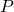is inductive.

Then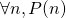.

This version still has a few words in it, but we could make it purely symbolic: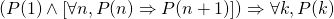To see that this version is the same as the wordier version we gave before, let's taketo be "can be reached from 1 by a sequence of successions". It's clear that thisis inductive: ifcan be reached from 1 by successions, so can its successor. Clearly 1 can be reached from 1 by a(n extremely short) sequence of successions. Someets both criteria -- the Inductive Axiom says "any natural number can be reached from 1 by a sequence of successions".

The Inductive Axiom is also known as the Principle of Mathematical Induction, or PMI for short. It's the engine that will let us prove lots of statements of the form, because that's the conclusion of PMI.

To use PMI in this way, you need to a few things:

• identify whatis
• verify thatis true (we call this the "base case")
• show thatis inductive, which means to prove(we call this the "inductive step")

Luckily, we already know how to prove universal claims, and we already know how to prove conditionals. So a general outline of a proof by induction goes like this:

Proof. (by induction)
base case: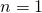. [Establish]

inductive step: Letbe a natural number. Assumeis true.
[. . . some work goes here . . .] Conclude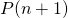.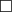Let's write an example proof by induction to show how this outline works. I'll put my commentary in blue parentheses.

Claim. I can reach any rung of any ladder which is standing on the ground.

Proof. Given a ladder, we'll show that for any, I can reach the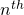rung of that ladder. (Hereis the statement "I can reach therung of the ladder.")

I can reach the first rung by taking a step -- after all, the ladder is standing on the ground. (We've just proved.)

Now suppose I'm standing on therung. (This is assuming.) Then I can take a step (These are the "juicy bits" of the proof.), and get to the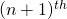step. (We've concluded.)

By the Principle of Mathematical Induction, this shows we can reach any rung of the ladder.Here's an example whereis "more mathematical".

Claim. For any natural number,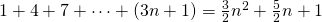.

Proof. (base case) When, the left-hand side is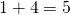. The right-hand side is. So the equality is true when.

(inductive step) Letbe a natural number. Assume. Consider the left-hand side of: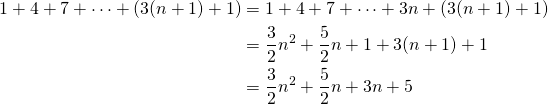Here we have used the assumption.

Now we work on the right-hand side of: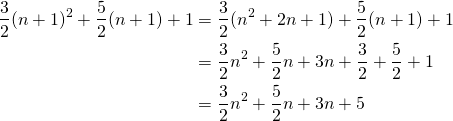Since the left-hand side ofand the right-hand side ofare both equal to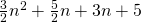, they are equal to each other. That is,is true. This completes the inductive step.

By the Principle of Mathematical Induction, we have established the claim..

A few things to note here. First, the base case is usually pretty obvious. Second, the fun (i.e. hard) part of the proof is figuring out how to useto get.

It's not always obvious what the natural number (or inductive variable) is. Often it's the size of something (since the natural numbers are for counting!). If your inductive variable isn't obvious from the problem statement, it's good form to let the reader know what you'll be inducting on.

Claim. Every finite set of real numbers has a least element.

Proof. We will proceed by induction on the size of the set.

(base case) Suppose the set has exactly one element. Then that element is least.

(inductive step) Assume we've proved that any set of real numbers with exactlydistinct elements has a least element. We'll show that any set of real numbers with exactly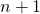distinct elements has a least element.

Let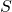be such a set, and label its elements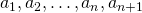. There are two possibilities for how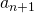is related to the other elements of the set.

case: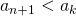for all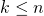. Thenis least.

case: There is somewith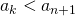. Then consider the set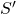which consists of all of the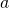s except.has exactlyelements, so by our inductive assumption, there is a least element of(call it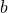). Then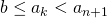, sois also least in.

In either case,has a least element, thus completing the inductive step, hence (by PMI) the proof.### "Generalized" induction

Now consider the following:

Claim. If I am standing in a second story window, I can get to any rung of any ladder standing on the ground, provided that rung is at least the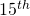rung.

The form of this claim is: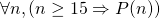, or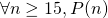.

Proof. Since I'm in the second story window, I can step out onto therung. (Prove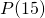.)

Now if I'm on any rung, I can take a step to get to the next rung. (Prove thatguarantees.)

So no matter what rung (above the) I want to get to, I can..

This technique of starting someplace other than 1 is sometimes called generalized induction, but it really doesn't deserve such a fancy name. It's just regular induction, but starting from some number other than 1.